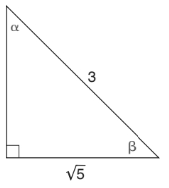Chapter 11.1, Problem 4E### Elementary Geometry for College St...

6th Edition
Daniel C. Alexander + 1 other
ISBN: 9781285195698

#### Solutions

Chapter
Section### Elementary Geometry for College St...

6th Edition
Daniel C. Alexander + 1 other
ISBN: 9781285195698
Textbook Problem
1 views

# In Exercises 1 to 6, find sin     α and sin     β for the triangle shown.To determine

To find:

The angle sinαandsinβ

Explanation

In a right angle triangle,

1) The side PQ, which is opposite to the right angle PRQ is called the hypotenuse.

(The hypotenuse is the longest side of the right triangle.)

2) The side RQ is called the adjacent side of angle θ.

3) The side PR is called the opposite side of angle θ.

Hence, the sine ratio for an acute angle is oppositehypotenuse

Given:

By using Pythagoras theorem, that is

c2=a2+b2

Let a be the opposite of the triangle.

From, the diagram we have c=3andb=5

32=a2+(5)29=a2+

### Still sussing out bartleby?

Check out a sample textbook solution.

See a sample solution

#### The Solution to Your Study Problems

Bartleby provides explanations to thousands of textbook problems written by our experts, many with advanced degrees!

Get Started

#### Sketch the graphs of the equations in Exercises 512. xy=x2+1

Finite Mathematics and Applied Calculus (MindTap Course List)

#### If f(x) exists and is nonzero for all x, then f(1) f(0).

Single Variable Calculus: Early Transcendentals, Volume I

#### Find for .

Study Guide for Stewart's Multivariable Calculus, 8th

#### Domain Find the value of c such that the domain of f(x)=cx2 is [5,5]

Calculus: Early Transcendental Functions (MindTap Course List)

#### True or False: 23xdx is an improper integral.

Study Guide for Stewart's Single Variable Calculus: Early Transcendentals, 8th